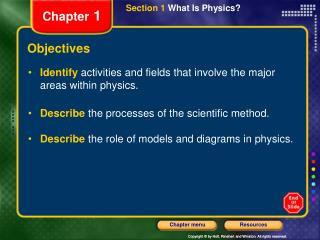# Objectives - PowerPoint PPT PresentationDownload PresentationObjectives

Download Presentation## Objectives

- - - - - - - - - - - - - - - - - - - - - - - - - - - E N D - - - - - - - - - - - - - - - - - - - - - - - - - - -
##### Presentation Transcript

1. Section 1 What Is Physics? Chapter 1 Objectives • Identifyactivities and fields that involve the major areas within physics. • Describethe processes of the scientific method. • Describethe role of models and diagrams in physics.

2. What is Physics? Physics is understanding the behavior and structure of matter Physics is a way of describing the natural world It deals with how and why matter and energy act as they do

3. Section 1 What Is Physics? Chapter 1 The Branches of Physics

4. Section 1 What Is Physics? Chapter 1 The Branches of Physics

5. Section 1 What Is Physics? Chapter 1 Physics • The goal of physicsis to use a small number of basic concepts, equations,and assumptions to describe the physical world. • These physics principles can then be used to makepredictions about a broad range of phenomena. • Physics discoveries often turn out to have unexpected practical applications, and advances in technology can in turn lead to new physics discoveries.

6. Section 1 What Is Physics? Chapter 1 Physics and Technology

7. Section 1 What Is Physics? Chapter 1 The Scientific Method • There is no single procedure that scientists follow in their work. However, there are certain steps common to all good scientific investigations. • These steps are called thescientific method.

8. Section 1 What Is Physics? Chapter 1 The Scientific Method

9. Section 1 What Is Physics? Chapter 1 The Scientific Method • Use thescientific method steps to investigate asking someone out on a date • Then check your plan by visiting • http://www.buildfreedom.com/content/scientific_method.shtml

10. Section 1 What Is Physics? Chapter 1 Models • Physics usesmodelsthat describe phenomena. • A modelis a pattern, plan, representation, or description designed to show the structure or workings of an object, system, or concept. • A set of particles or interacting components considered to be a distinct physical entity for the purpose of study is called asystem.

11. Section 1 What Is Physics? Chapter 1 Models

12. Section 1 What Is Physics? Chapter 1 Hypotheses • Models help scientists develophypotheses. • Ahypothesisis an explanation that is based on prior scientific research or observations and that can be tested. • The process of simplifying and modeling a situation can help you determine the relevant variables and identify a hypothesis for testing.

13. Section 1 What Is Physics? Chapter 1 Hypotheses, continued Galileo modeled the behavior of falling objects in order to develop a hypothesis about how objects fall. If heavier objects fell faster than slower ones,would two bricks of different masses tied together fall slower(b)or faster(c)than the heavy brick alone(a)?Because of this contradiction, Galileo hypothesized instead that all objects fall at the same rate, as in(d).

14. Section 1 What Is Physics? Chapter 1 Controlled Experiments • A hypothesis must be tested in acontrolled experiment. • A controlled experiment tests only one factor at a time by using a comparison of acontrol groupwith anexperimental group.

15. Section 1 What Is Physics? Chapter 1 Controlled Experiments

16. Section 1 What Is Physics? Chapter 1 Controlled Experiments

17. Section 1 What Is Physics? Chapter 1 Controlled Experiments You want to study the basketball and its motion based on observing its path through the air What information can you eliminate that is not important? • Write the following concerned with this situation • Research Question • Hypothesis • Experimental Design with Controlled Variables

18. Section 1 What Is Physics? Chapter 1 Research Question What properties of the thrown ball effects its path through the air? Hypothesis Factors that influence the position of the ball at any time are direction of throw, forces on the ball, spin on the ball, shape, size mass and air surrounding the ball Experimental Design with Controlled Variables Keep factors listed above constant but one of them i.e. vary amount of force on the ball keeping the angle of throw and the amount of spin constant. Note: Disregard ball’s color. A simple model might also determine that spin and size won’t have much effect so should be left out.

19. Section 1 What Is Physics? Chapter 1 What is a Scientific Theory? It is a large body of knowledge and well tested hypotheses about some aspect of the natural world What’s the difference between a Law and a Theory? A law is a hypothesis that has not so far been contradicted by experimental evidence. Theories often undergo change over time as more evidence is gathered. How does Science differ from Technology? Science is a method of answering theoretical questions while technology is a method of solving practical problems. Technology has to do with the tools, techniques and procedures for putting the findings (relationships) of science to use.